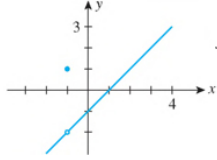Chapter 2.5, Problem 44E

Chapter
Section
Textbook Problem

In Exercises 39-44, determine the values of x, if any, at which each function is discontinuous. At each number where f is discontinuous, state the condition(s) for continuity that are violated.44.f ( x ) = { x 2 − 1 x + 1 if  x ≠ − 1 1 if  x = − 1

To determine

The value of x at which the function f(x)={x21x+1ifx11ifx=1 is discontinuous.

Explanation

Definition used:

The function will be continuous when the value of left hand limit and the value of right hand limit is equal to the value of the function at that point and value of that function should satisfy that point.

A function f is continuous at x=a when it follows the following three conditions,

(1) f(a) is defined.

(2) limxaf(x) exists.

(3) limxaf(x)=f(a).

Calculation:

The function is f(x)={x21x+1ifx11ifx=1 (1)

The graph of the function is shown below in Figure 1,

Figure (1)

Substitute 1 for x in equation (1) to find the left hand limit,

limx1f(x)=limx1(x21x+1)=limx1((x+1)(x1)x+1)((a2b2)=(a+b)(ab))=limx1(x1)(Canceloutthecommonfactor)=11

Further solve the above equation,

limx1f(x)=2

Substitute 1 for x

Still sussing out bartleby?

Check out a sample textbook solution.

See a sample solution

The Solution to Your Study Problems

Bartleby provides explanations to thousands of textbook problems written by our experts, many with advanced degrees!

Get Started

Show that the equation has exactly one real root. x3 + ex = 0

Single Variable Calculus: Early Transcendentals, Volume I

Solve the equation in problem 1-6.; 5.

Mathematical Applications for the Management, Life, and Social Sciences

In Exercises 1-10, determine which of the matrices are stochastic. [.8.2.3.7]

Finite Mathematics for the Managerial, Life, and Social Sciences